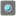• # 15 is maximum

## Question related to missionPearls in the Box

I'm sorry for the format, it's hard to paste.

```import re
def checkio(marbles, step):
b=marbles.count("b")
n=len(marbles)
for i in range(1,step+1):
if i==1:
c="(b-0)"
else:
pat='b'+(i-1)*'.\w'
s=re.findall(pat,c)
for i in s:
ti='('+i+')'+'*('+i+'-1)'+'+(n-('+i+'))*('+i+'+1)'
c=c.replace(i,ti)
#print(c)
d=1-eval(c)/(n**step)
d=round(d,2)
print(d)
return d
if __name__ == '__main__':
assert checkio("wwwwwwwwwwwwwwwwwwww",14) == 0.64, "1st example"
```

I want to use "re" to get formula in string， then use "eval" to get the result, but when the step bigger than 14, that will cost too much time... Is there any way to simplify it?19